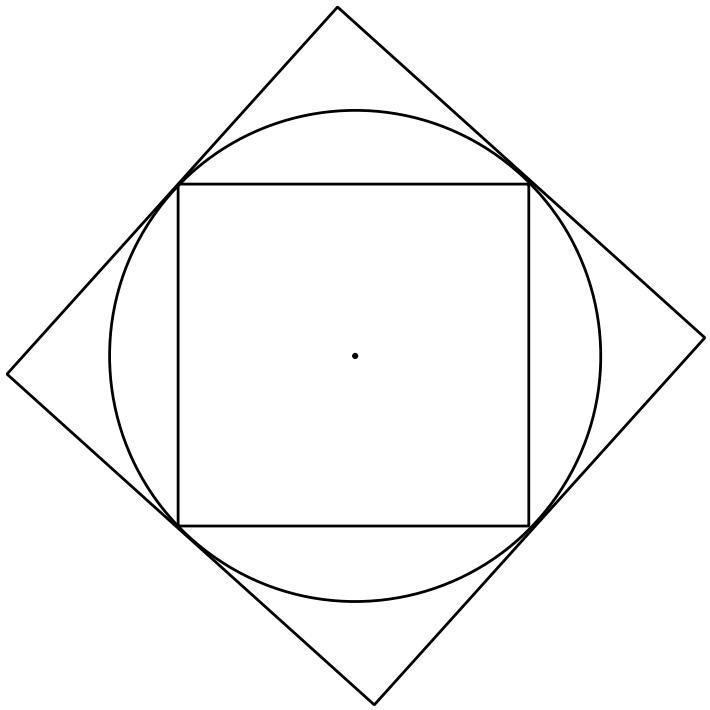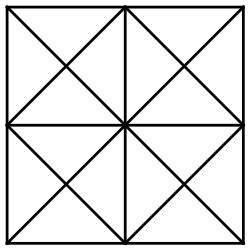# u3a - Maths Challenge – Week 8

## Maths Challenge – Week 8

• Published : 28 May 2020
• Views: 2933

Welcome to Week 8 of our weekly maths challenge, with problems and puzzles posed by Gordon Burgin, Andrew Holt and the U3A Maths and Stats Subject Adviser - David Martin.

If you would like to share your ideas on how to solve these puzzles please join our learning forum or discuss within your U3A and interest group. The solutions to week seven’s problems are at the bottom of the page. Check back each week for the solutions and let us know how you get on by contacting National Office. New maths puzzles will go up onto the website every Thursday.

### Week 8

Question 1
The figure below shows a square inside a circle which in turn lies inside a square. The inner square meets the outer square at its midpoints. What fraction of the area of the outer square is the area of the inner square.Question 2
The front wheel of a Penny Farthing bicycle has a diameter of 1.5m. This type of bicycle had no gears and had pedals attached to a large front wheel which was made as big as possible to increase speed. A cyclist pedals at 60 revolutions per minute. What speed will the cyclist be travelling at?

Question 3
111,111 is the product of 5 primes. What are they?

Question 4
3² + 4² = 5²
10² + 11² + 12² = 13² + 14²
21² + 22² + 23² + 24² = 25² + 26² + 27²
What is the next equation in this sequence?

### Week Seven Solutions

Question 1
I am a two-digit prime number having no divisors other than one and myself. One less than me is a square number while half of one more than me is also a prime number. What am I?

Solution
The two-digit square numbers in range are 16, 25, 36, 49, 64 and 81 which are one less than 17, 26, 37, 50, 65 and 82 respectively. 17 and 37 are the only primes. One half of one more than 17 is 9 which is not a prime number while one half of one more than 37 is 19 which is a prime number. The number is therefore 37 whose reversed digits 73 also form a prime number.

Question 2
Six years ago, Mikayla was 3 times as old as Tatum was. If Mikayla is now 24 years old, how old is Tatum?
Solution
Let Tatum’s age now be T years and Mikayla’s age now be M years.
Then (M - 6) = 3(T - 6) and M = 24
So 18 =3(T - 6)
6 = T – 6
T =12
Tatum is 12 years old.

Question 3
How many triangles of various sizes are in the figure?Solution
Listing the number of triangles in increasing size
16 + 16 + 4 + 4 = 40 triangles
Question 4.
James has more than 250 toy soldiers. When he tries to put them in rows of 3 there are 2 left over. When he tries to put them in rows of 5 there are 2 left over. When he tries to put them in rows of 7 there are 2 left over. What is the least number of toy soldiers that James may have?
Solution
Let N be the number of soldiers he has.
Then N = 3x+2 = 5y +2 = 7z + 2 for some whole numbers x, y, z.
N - 2 = 3x = 5y = 7z. But 3 ,5, 7 do not have any factors in common, they are coprime, so N – 2 = n x 3 x 5 x 7 = 105n for some n
The first such number above 250 is when n=3 giving N - 2 = 105x3 = 315 i.e. N =317